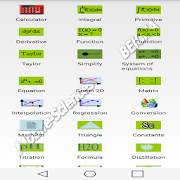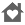# Mathematical & Chemical ToolsEveryone
4The recent version of MobileMaths contains many features and with user interface improved.

MobileMaths is a set of mathematical and chemical applications for Mobile Phones and Tablets. MobileMaths program includes a lot of applications with a large of features. It is designed in order to fulfil the needs of every one(student, engineer, researcher... .). By using MobileMaths you can make your handset as home computer and will get substantially all of the computing capabilities desired by a large fraction of the public. All the calculations made by MobileMaths are accurate. MobileMaths includes the following powerful and improved tools:

1. Professional Programming Calculator:
Programming statements are mostly compatible with Matlab. Similar to the Matlab's command window.

2. Periodic Table:

3. Function Solver

4. Equation Solver:
Solve any equations from linear to more complex ones.

5. Interpolation Solver:
1-D Cubic spline interpolation of x/y-values at xi, interpolates to find yi.

6. Graph 2D Solver:
You can plot many curves in a single graph. You can also opening and saving your data.

7. Matrix Solver:
Multiplication, A*B
Inverse of matrix
Determinant of matrix
Linear system solver, A*x=b
Key Features:
• Solving a linear equation system of up to 20 unknowns
• Flexible data entry
• Opening and saving data

8. Curve Fitting (Regression) Solver:
Non linear fitting tool enables you to perform curve fitting of one- dimensional data using arbitrary (non linear) fitting functions by computing the coefficients or parameters. The tool is based on Nelder-Mead method, which performs an unconstrained nonlinear minimization of the sum of squared residuals with respect to the various parameters. The tool is unique in its kind available for mobile phones and tablets.
Polynomial regression finds the coefficients of a polynomial p(x) of degree n that best fits the data in a least-squares sense.
Multiple linear regression attempts to model the relationship between two or more explanatory variables and a response variable by fitting a linear equation to observed data.

Key Features:
Linear & polynomial fitting
• Find the best-fit line or polynomial curve to fit your input data
• Multiplelinear regression up to 10 independant variables
• Flexible data entry
• Opening and saving data

Nonlinear fitting (Nonlinear Regression)
• User defined regression model (input function) can have many fitting parameters, or variables, and you can use whatever variable names you want to use in your input function.
• The tool implements the Nelder-Mead simplex (direct search) algorithm, to minimize a non linear function of several variables.
• Flexible data entry
• Opening and saving data

9. Derivatives Solver

10. Tool for simplifying expressions

11.Tool for solving systems of nonlinear equations of several variables

12. Definite Integral Solver

13. Indefinite Integral Solver

14. Triangle Solver

15. Titration Solver

16. Molecular Weight Solver

17. Programming Language MathPro:
MathPro is a numerical computing environment and programming language mostly compatible with Matlab. You can implement any numerical method using this program. MathPro is designed in order the user interact more efficiently. This Mathematical tool for Mobile Phones and Tablets is a Must-have tool for students, professionals and scientists. MathPro's interface integrate standard mathematical notation, programming statements, and text in a single worksheet. Is very flexible, fast, and more professional. Programming statements are mostly compatible with Matlab.

18. Taylor Series Calculator

19. Unit Converter

20. Distillation Solver

22. Solubility Solver

24. and much more...

MobileMaths has many features that are unique by their kind available for Android Phones and Tablets.

Supported languages included:
1. English
2. French
3. German
4. Italian
5. Spanish
6. Portuguese

Collapse

## Reviews

Review Policy
4.5
4 total
5
4
3
2
1

Eligible for Family LibraryLearn More
Updated
March 22, 2019
Size
12M
Installs
50+
Current Version
82.0
Requires Android
4.1 and up
Content Rating
Everyone
Permissions
Offered By
mobile-sciences
Developer
Rue AI no: 3 Bouakel 3 Batna 05000 Italie